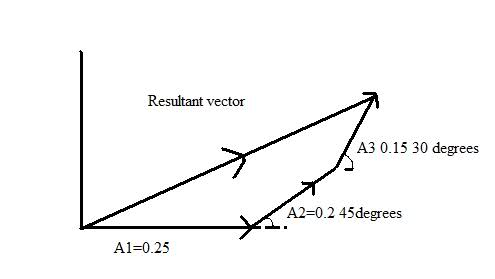# Resultant component of adding Three simple harmonic motions together help Diagram!

mmmboh
Hi, this isn't homework but a practice problem.
A particle is simultaneously subjected to three simple harmonic motions, all of the same frequency and in the x direction. The amplitudes are 0.25, 0.20, 0.15, and the face difference between the first and second is 450 and between the second and third is 300. Find the amplitude of the resultant displacement and its phase relative to the first component.The 0.25, 0.20, and 0.15 components are represented by Z1=A1ejwt, Z2=A2ej(wt+a), and Z3=A3ej(wt+b), adding them all together to give me the resultant component, and simplifying gives me: Z=ejwt[A1+A2eja+A3ejb]...so what I would think I am suppose to do is find the x component of the resultant vector and the y component, and use Pythagorean to find the resultant vector and then do arctan(y/x) to get the phase angle. The answers are 0.52 and 33.50.
I can get 0.52 for the amplitude, but that is only by taking the x component of the resultant vector and ignoring the rest, if I do it with Pythagorean I get [0.522 +0.2162]1/2=0.56, and the angle is arctan (0.216/0.52)=22.5......so apparently I am wrong, can someone help please?

willem2

I can get 0.52 for the amplitude, but that is only by taking the x component of the resultant vector and ignoring the rest, if I do it with Pythagorean I get [0.522 +0.2162]1/2=0.56, and the angle is arctan (0.216/0.52)=22.5......so apparently I am wrong, can someone help please?

I can only get 0.52 for the amplitude if I use both the x and the y component of the resultant vector. I also don't get 0.216 for the y component. If you want to know

mmmboh

Ok well for the x component I did 0.25+0.20cos45+0.15cos30=0.52...and for the y component I did 0.20sin45+0.15sin30=0.216...what am I doing wrong?

mmmboh

Hm I guess if i use 75 degrees for the third one instead of 30 degrees it works, that's how I originally did it, but in the book they made it seem like that's not the way :S

Edit: I think I see my mistake